# Fahrenheit To Celsius Formula, Charts and Conversion

By Nick Valentine| Last update: 17 June 2017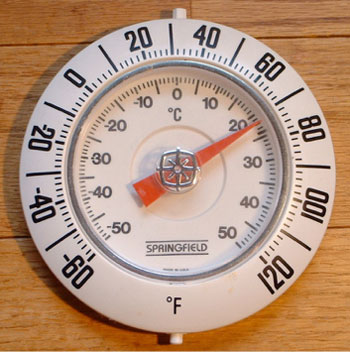In this article, we will show you the easiest and most accurate ways to convert temperatures from Fahrenheit to Celsius and from Celsius to Fahrenheit.

At first glance, the two scales do not seem to relate to each other at all and this is because of the different methodologies of the scientists after which they are named.

As a note, before you start reading. You will find, on the second page of this article, some handy reference charts and a converter, to allow you to quickly convert between the units. Quick links to those are included below.

## The Fahrenheit scaleThe German physicist Daniel Gabriel Fahrenheit (1686 - 1736) came first. In 1714, he invented the mercury-in-glass thermometer that 300 years on is still one of the most accurate and most widely used means of measuring temperature.

Ten years later, Fahrenheit first proposed his temperature scale that set freezing point at 32 degrees (32°F) and water boiling point at 212°F.

Why these points? For a start, Fahrenheit realised that the temperature at which water starts to freeze is a fair bit warmer than the coldest temperature measurable, which he calibrated by placing a thermometer into a mixture of iced water and ammonium chloride salt. This formed his zero point.

He then observed that the temperature at which ice starts to form on the surface of water was 32 degrees warmer than zero. His third reference point was human body temperature (measured by placing the thermometer in the mouth or under the arm), which he calibrated at 96 degrees (later refined to 98.6°F)

Fahrenheit's theoretical ultimate temperature was the boiling point of mercury, calculated at 600 degrees. It was work by others that showed that on Fahrenheit's scale, the boiling point of water is about 179 degrees above its freezing point and this was standardised to 180 degrees, which is a nicely rounded (composite) number that is divisible by 2, 3, 4, 5, 6, 8, 9, and 10.

## The Celsius scaleThe year Fahrenheit published his original scale, young Anders Celsius (1701 - 1744) was a budding physicist, astronomer and mathematician. He went on to found Uppsala Astronomical Observatory in his native Sweden and a year later, in 1742, proposed his own temperature scale.

By this point, Celsius had done a lot of research on temperature observing, for instance, that the boiling point of water varies with barometric pressure.

Since he was aiming for a scale that could be used worldwide, Celsius set water boiling and freezing points exactly 100 degrees apart, calling his scale Centigrade from the Latin for "100 steps". Eccentrically, Celsius set freezing point at 100°C and water boiling point at 0°C. After the Great Man's death, fellow Swede Carl Linnaeus suggested to the Swedish Academy that this be reversed and a more intuitive scale was adopted in 1745.

## Kelvin scale

We should also note that most scientists and engineers would not use either of these scales but would prefer a third one, the kelvin system developed by British physicist William Thomson, Baron Kelvin. This is based on recognition that heat is actually caused by movement of molecules inside a substance, with a theoretical Absolute Zero point at which there is no molecular activity at all. Just how cold this is can be understood when you note that on the Kelvin scale, water freezing (actually called the 'triple point' at which water, ice and water vapour can all co-exist) occurs at 273.16K, Boiling point is set exactly 100 points higher at 373.16K. Thus the kelvin represents the same magnitude of heat as the degree Celsius.

## Switching from Fahrenheit to Celsius

From the mid-18th to mid-20th centuries, Fahrenheit was the system most widely used in English-speaking countries, while continental Europeans preferred 'The Swedish Scale'. With the drive to standardisation and metrication from the 1950s, Celsius became the adopted temperature scale for the EEC (later EU), with the UK and Ireland converting (somewhat reluctantly) after joining the European community.Is it 88°F or 31.1°C? You decide...

This reluctance was partly due to human preference. For while the Celsius scale was developed for convenience, Fahrenheit's scale was based on observation and measurement. Also, whereas Fahrenheit had developed a ratio scale, Celsius had produced an interval scale. These are very different things, as we shall explain in a future article.

This may help explain why many people prefer Fahrenheit readings as rather closer to actual experience. In Celsius, a chilly day at 11°C does not seem greatly different from a really hot one at 35°C. On the Fahrenheit scale, these respectively measure at about 52°F and 95°F, with the latter traditionally taken as license for red top tabloids to start running 48 point headlines containing words like 'Phew', 'Flaming' and 'Scorcher'.

Incidentally, while the UK's Met Office started publishing temperatures in both Celsius and Fahrenheit from 1962 and dropping use of Fahrenheit altogether from official reports in 1970, British media persisted in using Fahrenheit in weather reports well into the 21st century. Indeed it became the fashion to use Celsius when describing extreme winter conditions and Fahrenheit for heat waves - a nice example of double standards in action.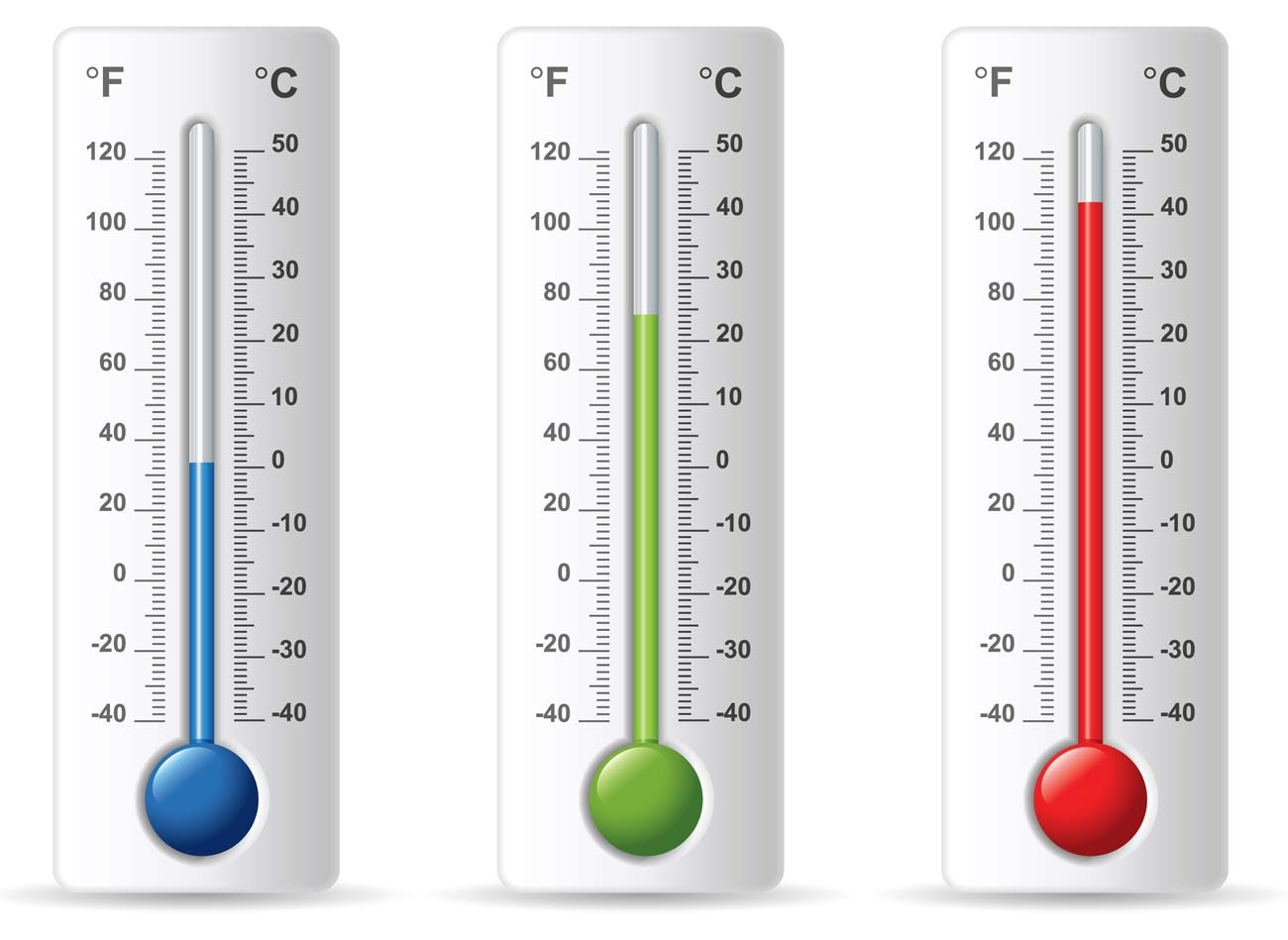## Fahrenheit and Celsius Formula

But how would you be able to convert those temperatures for yourself without access to one of those widgets on your phone or laptop?

It is made more complicated by that difference between freezing points, 32°F versus 0°C. From there on up to boiling, 180 degrees in Fahrenheit are equivalent to 100 degrees Celsius.

### Official conversion formula

The formula to convert Fahrenheit to Celsius is:

Fahrenheit to Celsius Formula:

[°C] = ([°F] - 32) × 5/9

In other words, take your Fahrenheit reading, subtract 32, multiply the answer by five and then divide by nine. So 66 degrees Fahrenheit minus 32 would be 34, times five makes 170 divided by nine equals 18.88 recurring, or 18.9°C.

To go the other way, from Celsius to Fahrenheit:

Celsius to Fahrenheit Formula:

[°F] = [°C] × 9/5 + 32

Here, you multiply your Celsius temperature by nine, divide by five and then add 32 to find the Fahrenheit reading.

So, 13 degrees Celsius times nine is 117, divided by five is 23.4, plus 32 makes 55.4°F.

You can see where the problem is in both these sums; it's that nine.

## Easier methods of conversion

There's a rough and ready way round this, based on 9/5 being close to doubling and 5/9 being near half. Under this system, double your Centigrade and add 32 to find Fahrenheit.

Alternatively, take Fahrenheit, subtract 32 and halve the answer to get to Celsius.

Under this system, that 66°F would convert to 17°C, nearly two degrees lower than the correct answer while the 13°C would convert to 58°F, which is close to three degrees higher than the true figure.

We're left with an uncomfortable choice between cumbersome sums and distinctly dodgy accuracy: is there any way to work around this dilemma?

## The Rule of 40

For a start, we can use 40 instead of 32. This is because it so happens that -40°F is also -40°C. So under this system to go from Fahrenheit to Celsius, you add 40, multiply by 5, divide by 9, then subtract 40. Going from Celsius to Fahrenheit you also add 40 to the centigrade reading, multiply by nine, divide by 5, then subtract 40.

It may sound strange but it does indeed work, like this:

Our original 66 degrees Fahrenheit plus 40 makes 106, times five equals 530, divided by nine gives us 58.88 recurring, rounding up to 58.9, minus 40 takes us once again to 18.9°C.

And working the other way, from Celsius to Fahrenheit:

That 13 degrees Celsius plus 40 gives 53, which multiplied by nine makes 477, divided by five to make 95.4, minus 40 gives us, once again, 55.4°F.

## Using decimals

There's one last thing we can do to make life a little simpler, using decimals instead of fractions. That means using 1.8 instead of 9/5.

This gives modified formulae of:

• Celsius to Fahrenheit:
(°C + 40) × 1.8 - 40 = °F
• Fahrenheit to Celsius:
(°F + 40) / 1.8 - 40 = °C

Trying this out in action on a hot day (and remembering that multiplying by 1.8 is the same as doubling and then subtracting 10 per cent) we could have 36°C plus 40 is 76, times 1.8 is 136.8 minus 40 is 96.8°F, which is firmly in 'Scorcha' territory.

And the other way, on a rather chillier day, 48°F plus 40 is 88, divided by 1.8 is 48.88 recurring (48.9) minus 40 equals 8.9°C, which actually does sound quite cold.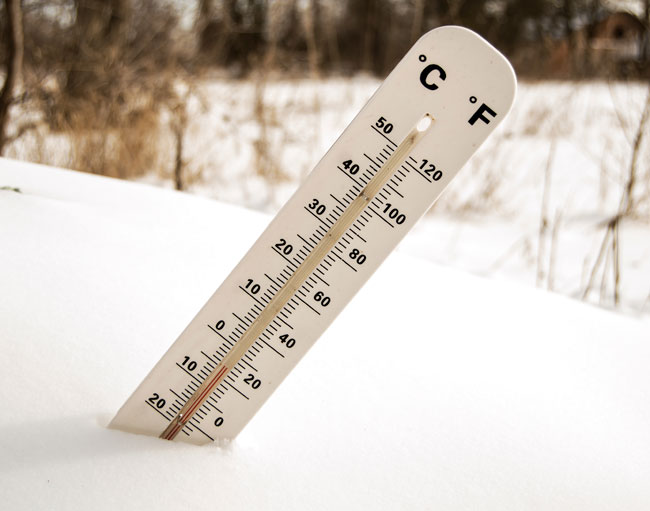It always looks chillier in Celsius...

## Mental reference points

In actual life, the best way might be to fix some reference points in your mind:

1. A genuine heat wave day is 95°F and that's 35°C.
2. Temperatures over 40°C (105°F) are dangerous, with real risk of heat stroke or dehydration. Stay in the shade and drink plenty of water.
3. A really chilly take-your-coat-and-gloves-and-don't-forget-your-woolly-hat-and-scarf day is around 2°C; a little under 36°F.
4. The temperature that most of us like to lounge around in at home is 70 degrees Fahrenheit, so set the central heating thermostat to 20°C. If you're in the air conditioning zone, then the numbers are 75°F or 24°C.
5. On a health note, anything over 103°F (39.4°C) constitutes a dangerous fever. If the patient's temperature reaches this point, you need urgent medical assistance.

## Reference Chart 1: Fahrenheit to Celsius

Celsius readings rounded up or down to single decimal points.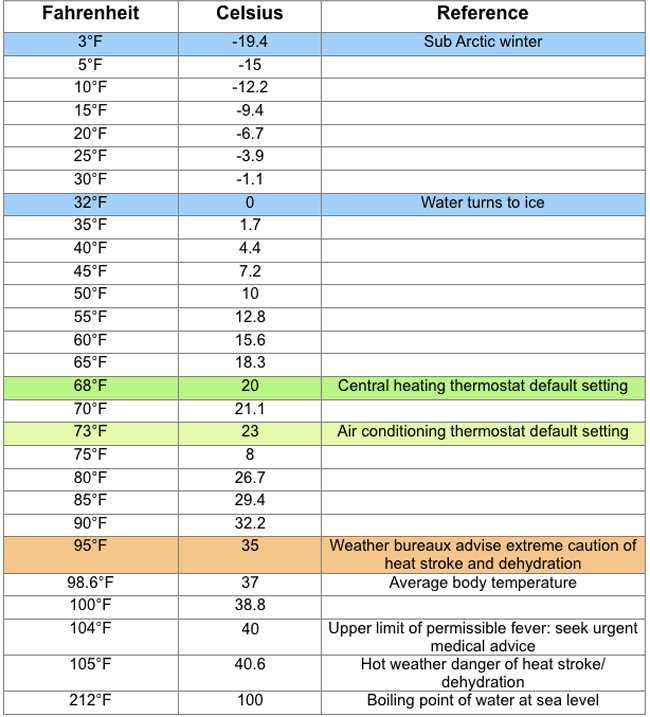## Reference Chart 2: Celsius to Fahrenheit

Fahrenheit readings rounded up or down to nearest whole degree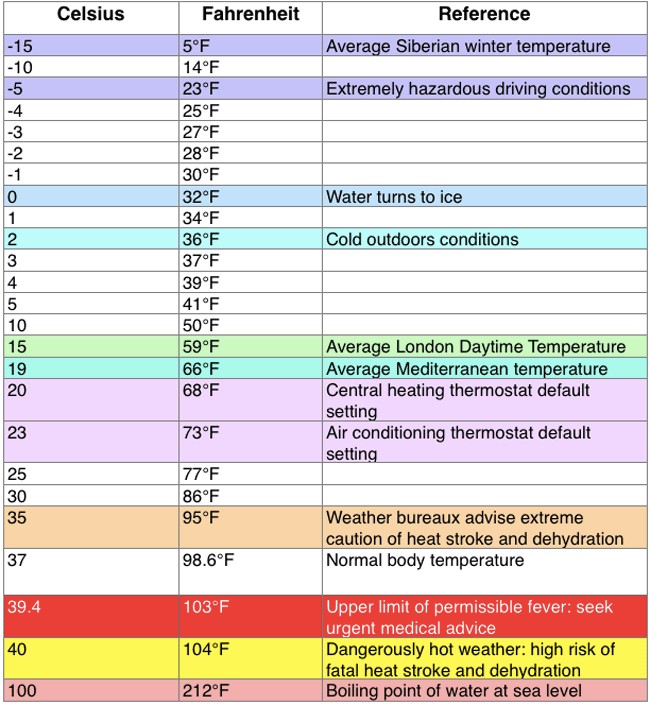Of course, if you're looking for a pain-free method of doing the calculation right here and now then you can use our handy Fahrenheit and Celsius converter, below.

## Fahrenheit and Celsius Converter

Our website provides a comprehensive list of online conversion tools. So, should you wish to convert between fahrenheit, celsius and any other units of temperature, give the temperature converter a try.